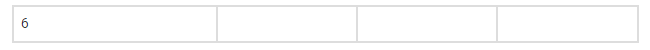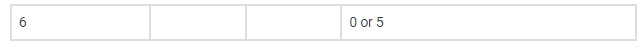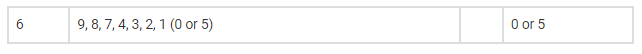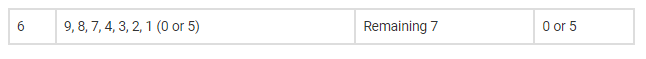# Find the number of positive integers greater`
Question:

Find the number of positive integers greater than 6000 and less than 7000 which are divisible by 5, provided that no digit is to be repeated.

Solution:

(i)Thousand’s Place can be fill with 6 alone.

Hence, number of way =1Unit place can be filled with either 0 or 5.

Hence, number of way =2Hundred’s place can be filled with the remaining 8 digits.

Hence, number of way =8Ten’s place can be filled with 7 digits.

Number of ways= 7.Thus, required number will be

=1 x 8 x 7 x 2

=112